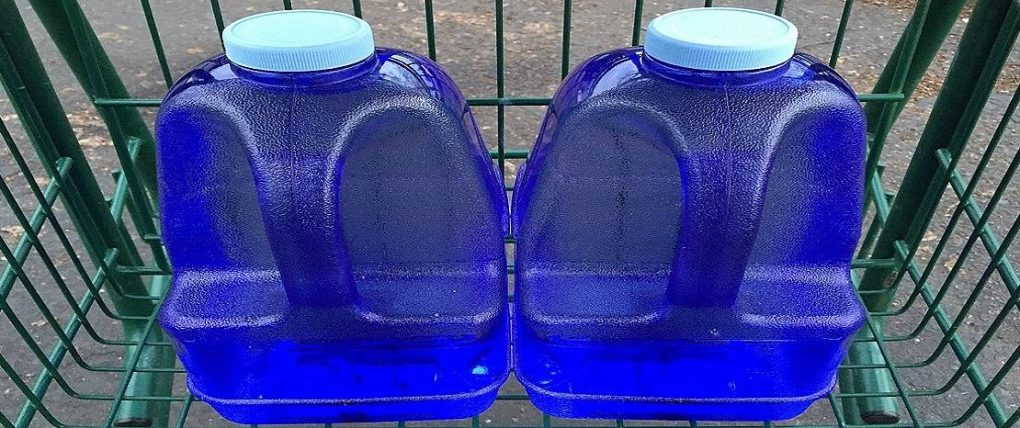# How many ounces in a gallon?When we ask the question “How many ounces in a gallon?” we are, of course, referring to fluid ounces. That is, the ounce that is used as a measure of the volume of a liquid as opposed to that which is used as a measure of the mass of something. But just how many fluid ounces are in a gallon?

## How many ounces in a gallon?

To answer this question, we need to know which definition of an ounce we are using. This is because over time there have been various different definitions of the fluid ounce. Only two remain in common use today, though. They are the British imperial fluid ounce and that used by the United States customary measurement system.

So let’s take a look at each of these in turn and see how many fluid ounces are in each of these measurement system’s gallons…

### British imperial system

The imperial fluid ounce is one twentieth of an imperial pint. As there are eight imperial pints in a gallon, this means that there are 160 imperial fluid ounces in an imperial gallon.

1 imperial gallon = 160 imperial fluid ounces.

### U.S. customary system

The U.S. customary fluid ounce is one sixteenth of a U.S. customary pint. As with the imperial measurement system. there are eight U.S. pints in a U.S. gallon. So this means that there are 128 U.S. fluid ounces in a U.S. gallon.

1 U.S. gallon = 128 U.S. fluid ounces.